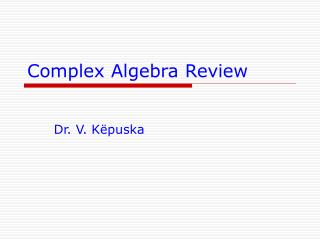Download PresentationComplex Algebra Review

# Complex Algebra Review - PowerPoint PPT Presentation

Complex Algebra Review. Dr. V. K ëpuska. Complex Algebra Elements. Definitions: Note: Real numbers can be thought of as complex numbers with imaginary part equal to zero. Complex Algebra Elements. Euler’s Identity. Polar Form of Complex Numbers.I am the owner, or an agent authorized to act on behalf of the owner, of the copyrighted work described.
Download Presentation## Complex Algebra Review

An Image/Link below is provided (as is) to download presentation

Download Policy: Content on the Website is provided to you AS IS for your information and personal use and may not be sold / licensed / shared on other websites without getting consent from its author.While downloading, if for some reason you are not able to download a presentation, the publisher may have deleted the file from their server.

- - - - - - - - - - - - - - - - - - - - - - - - - - E N D - - - - - - - - - - - - - - - - - - - - - - - - - -
Presentation Transcript
1. Complex Algebra Review Dr. V. Këpuska

2. Complex Algebra Elements • Definitions: • Note: Real numbers can be thought of as complex numbers with imaginary part equal to zero. Veton Këpuska

3. Complex Algebra Elements Veton Këpuska

4. Euler’s Identity Veton Këpuska

5. Polar Form of Complex Numbers • Magnitude of a complex number z is a generalization of the absolute value function/operator for real numbers. It is buy definition always non-negative. Veton Këpuska

6. Polar Form of Complex Numbers • Conversion between polar and rectangular (Cartesian) forms. • For z=0+j0; called “complex zero” one can not define arg(0+j0). Why? Veton Këpuska

7. Geometric Representation of Complex Numbers. Axis of Imaginaries Im Q2 Q1 z Axis of Reals |z| Im{z}  Re{z} Re Q3 Q4 Complex or Gaussian plane Veton Këpuska

8. Geometric Representation of Complex Numbers. Axis of Imaginaries Im Q2 Q1 z Axis of Reals |z| Im{z}  Re{z} Re Q3 Q4 Complex or Gaussian plane Veton Këpuska

9. Im z1 1 z2 -2 -1 Re z3 -1 Example Veton Këpuska

10. Conjugation of Complex Numbers • Definition: If z = x+jy∈ C then z* = x-jy is called the “Complex Conjugate” number of z. • Example: If z=ej (polar form) then what is z* also in polar form? If z=rej then z*=re-j Veton Këpuska

11. Geometric Representation of Conjugate Numbers • If z=rej then z*=re-j Im z y r  x Re - r -y z* Complex or Gaussian plane Veton Këpuska

12. Complex Number Operations • Extension of Operations for Real Numbers • When adding/subtracting complex numbers it is most convenient to use Cartesian form. • When multiplying/dividing complex numbers it is most convenient to use Polar form. Veton Këpuska

13. Addition/Subtraction of Complex Numbers Veton Këpuska

14. Multiplication/Division of Complex Numbers Veton Këpuska

15. Useful Identities • z ∈ C,  ∈ R & n ∈ Z (integer set) Veton Këpuska

16. Useful Identities • Example: z = +j0 • =2 then arg(2)=0 • =-2 then arg(-2)= Im j  z -2 -1 0 1 2 Re Veton Këpuska

17. Silly Examples and Tricks Im j  /2 -1 0 3/2 1 Re -j Veton Këpuska

18. Division Example • Division of two complex numbers in rectangular form. Veton Këpuska

19. Roots of Unity • Regard the equation: zN-1=0, where z ∈ C & N ∈ Z+ (i.e. N>0) • The fundamental theorem of algebra (Gauss) states that an Nth degree algebraic equation has N roots (not necessarily distinct). • Example: • N=3; z3-1=0  z3=1 ⇒ Roots of Unity NextSlide Veton Këpuska

20. Roots of Unity • zN-1=0 has roots z=k, k=0,1,..,N-1, where • The roots ofare called Nthroots of unity. Veton Këpuska

21. Roots of Unity • Verification: Veton Këpuska

22. Geometric Representation Im j1 J1 2/3 J0 4/3 2/3 -1 0 1 Re 2/3 J2 -j1 Veton Këpuska

23. Important Observations • Magnitude of each root are equal to 1. Thus, the Nth roots of unity are located on the unit circle. (Unit circle is a circle on the complex plane with radius of 1). • The difference in angle between two consecutive roots is 2/N. • The roots, if complex, appear in complex-conjugate pairs. For example for N=3, (J1)*=J2. In general the following property holds: JN-k=(Jk)* Veton Këpuska